# Excel TEXT function with formula examples

This tutorial explains the specificities of the Excel TEXT function and shows some clever ways to use Text formulas in Excel.

Microsoft Excel is all about numbers, and in your worksheets, you are likely to deal with numbers most of the time. However, there are situations when you need to convert numbers to text, and it is where the TEXT function steps in.

## Excel TEXT function - syntax and basic uses

In its pure essence, TEXT in Excel is used to convert a numeric value to a text string in a specific format.

The syntax for the Excel TEXT function is as follows:

TEXT(value, format_text)

Where:

• Value - the numeric value to be converted to text. It can be a number, date, reference to a cell containing a numeric value or another function that returns a number or date.
• Format_text - the format that you want to apply. It is supplied in the form of a format code enclosed in the quotation marks, e.g. "mm/dd/yy".

The TEXT function is available in all versions of Excel 365, Excel 2021, Excel 2019, Excel 2016, Excel 2013, Excel 2010, Excel 2007, and lower.

Generally, an Excel TEXT formula is used in the following situations:

• To display numbers in a more readable way or in a format that makes more sense for your users.
• To display dates in a specific format.
• To combine numbers or dates with certain text or characters.

For example, if you want to pull the date from cell A2 and show it in another cell in the traditional date format like "January 1, 2016", you use the following Excel TEXT formula:

`=TEXT(A2, "mmmm d, yyyy")`

The result will look similar to this: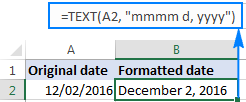Important note! The TEXT function in Excel converts a numeric value to a text strings. Consequently, you won't be able to use the result of your Text formula in other calculations. If there's such a need, you can keep the original values (hidden or kept out of sight), and use them in other formulas.

## Excel TEXT function format codes

As you have just seen, the syntax of the Excel TEXT function is very straightforward, a tricky part is supplying a proper format code that will output your number the way you want. Essentially, the TEXT function accepts most of the format codes used in Excel number formats. The table below lists the most common and frequently used ones.

 Code Description Format code example 0 Digit placeholder that displays insignificant zeros. #.00 - always displays 2 decimal places. If you type 2.5 in the referenced cell, it will display as 2.50. # Digit placeholder that does not display extra zeros. #.## - displays up to 2 decimal places. If you type 2.5, it will display as 2.5. If you type 2.555, it will display as 2.56. ? Digit placeholder that leaves a space for insignificant zeros but doesn't display them. It is generally used to align numbers in a column at a decimal point. #.?? - displays a maximum of 2 decimal places and aligns the decimal points in a column. . (period) Decimal point , (comma) Thousands separator. ###,###.## - displays a thousands separator and 2 decimal places. If you type 250000, it will display as 250,000.00

Additionally, you can include any of the following characters in the format code, and they will be displayed exactly as entered.

 Symbol Description + and - Plus and minus signs ( ) Left and right parenthesis : Colon ^ Caret ' Apostrophe { } Curly brackets < > Less-than and greater than signs = Equal sign / Forward slash ! Exclamation point & Ampersand ~ Tilde Space character

The following spreadsheet shows how you can use a Text formula in Excel to apply different formatting types to the same value.

A B C
1 Original Value Formatted Value Formula
2 5.5 5.50 =TEXT(A2, "0.00")
3   550% =TEXT(A2,"#%")
4   \$5.50 =TEXT(A2, "\$#,##0.00")
5   + \$5.50 =TEXT(A2, "+ \$#,##0.00;- \$#,##0.00;\$0.00")
6   - \$5.50 =TEXT(A2, "- \$#,##0.00;- \$#,##0.00;\$0.00")
7   5 1/2 =TEXT(A2,"# ?/?")
8   5.50E+00 =TEXT(A2,"0.00E+00")
9   ~6 ! =TEXT(A2,"~# !")

When using the Excel TEXT function with dates and times, you can use any of the following format codes.

 Format code Description Examples d Day of month, or day of week d - one or two-digit number without a leading zero (1 to 31) dd - two-digit number with a leading zero (01 to 31) ddd - three-letter abbreviation (Mon to Sun) dddd - full name of day of week (Monday to Sunday) m Month (when used as part of a date) m - one or two-digit number without a leading zero (1 to 12) mm - two-digit number with a leading zero (01 to 12) mmm - abbreviated month (Jan to Dec) mmmm - full name of month (January to December) y Year yy - two-digit number (e.g. 06 meaning 2006 or 16 meaning 2016) yyyy - four digit number (e.g. 2006, 2016) h Hour h - one or two-digit number without a leading zero (1 to 24) hh - two-digit number with a leading zero (01 to 24) m Minute (when used as part of time) m - one or two-digit number without a leading zero (1 to 60) mm - two-digit number with a leading zero (01 to 60) s Second s - one or two-digit number without a leading zero (1 to 60) ss - two-digit number with a leading zero (01 to 60) AM/PM Time represented as a 12-hour clock, followed by "AM" or "PM"

The following spreadsheet shows a few Excel Text formulas for dates:

A B C
1 Original Date Formatted Date Formula
2 12/09/2016 Dec 09 2016 =TEXT(A2, "mmm dd yyyy")
3   Friday 09 December, 2016 =TEXT(A2, "dddd dd mmmm, yyyy")
4   9-Dec-16 =TEXT(A2, "d-mmm-yy")
5   Friday =TEXT(A2,"dddd")

## How to use TEXT function in Excel - formula examples

So far, you might have an impression that the use of the Excel TEXT function is quite limited (because a value's display can easily be changed using the Format Cells dialog available via a cell's context menu or Ctrl+1 shortcut). The situation changes as soon as you start using TEXT in combination with other Excel functions. The below examples will give you a few inspirational ideas.

### Concatenate text and number (or date) in a custom format

When creating summary sheets or reports, you may often want not only calculate totals, percentages and the like, but also explain to your users what this or that number means. To do this, use the CONCATENATE function to join text and numbers, and the TEXT function to display the number (or date) like you want it.

#### Example 1. Format numbers inside a text string

Supposing you calculate the total amount based on the unit price in cell A2, quantity in B2, and discount percentage in C2 using this calculation: `=A2*B2*(1-C2)`. For your users to know exactly what the output number means, you wish to display it together with some explanatory text like "Your price is". Additionally, you want to show the currency symbol, thousands separator and two decimal places.

To have it done, supply the above calculation in the 1st argument of the TEXT function, include the corresponding format code in the 2nd argument, and concatenate the Text formula with a string using either the ampersand operator or CONCATENATE function:

`="Your price is "&TEXT(A2*B2*(1-C2), "\$###,###.00")`

or

`= CONCATENATE("Your price is ",TEXT(A2*B2*(1-C2), "\$###,###.00"))`

The following screenshot demonstrates the result: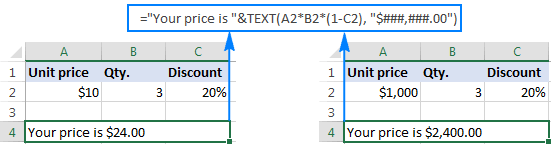#### Example 2. Combine text and date in the desired format

When you return the current date using TODAY() or NOW() function, you may also want to display it in combination with some text so that no one has any doubt what day this date refers to.

However, if you try to concatenate a text and date in the usual way:

`=CONCATENATE("Today is ", TODAY())`

Excel will return a very odd result, something like "Today is 42198".

The point is that in the internal Excel system, dates are stored as numbers, and that number appears in a concatenated text string. To fix this, use the TEXT function to display the date the way you want.

For example, embedding the TODAY function in the Text formula with the format code "dddd d mmm, yyyy" will return a string similar to this: "Today is Monday 12 Dec, 2016".

The complete formula goes as follows:

`=CONCATENATE("Today is ", TEXT(TODAY(), "dddd d mmm, yyyy"))`

or

`="Today is " & TEXT(TODAY(), "dddd d mmm, yyyy")`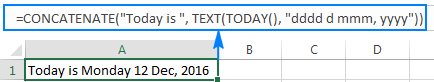A few more formula examples can be found here: Concatenate numbers and dates in various formats.

As you know, Microsoft Excel automatically removes leading zeros typed before a number in a cell, which works fine in most situations. But what if you want to keep the preceding zeros?

The Excel TEXT function can be an easy solution to pad numbers with leading zeros in a column, even if the original values are not the same length. Simply use the format code containing only zero placeholders like "0000000", where the number of zeros corresponds to the number of digits you want to display.

For example, to display 7-digit numbers with leading zeros, use this formula (where A2 is the original number):

`=TEXT(A2,"0000000")`

As you can see in the screenshot below, our Excel Text formula adds as many leading zeros as necessary to make a 7-character long string (please remember, the result of the TEXT function in Excel is always a text string, even if it looks like a number).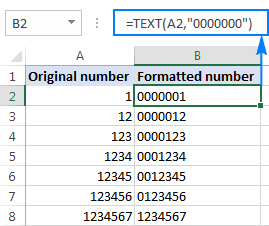### Convert values to phone numbers in a specific format

Turning a column of numeric values into telephone numbers may sound like a tricky task, but only until you remember that the Excel TEXT function allows using dashes and parentheses in format codes.

So, to display a number in A2 in a traditional US local 7-digit phone format like 123-456, use this formula:

`=TEXT(A2, "###-####")`

If some of the original values may contain a domestic prefix (i.e. there can be both 7-digit or 10-digit numbers), include the following conditional format code to display 10-digit numbers in the (123) 456-789 format:

`=TEXT(A2,"[<=9999999]###-####;(###) ###-####")`

The screenshot below shows this Excel Text formula in action: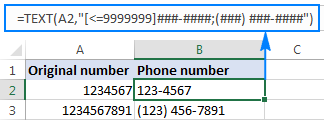## Excel TEXT function not working - reasons and solutions

Compared to other Excel functions, TEXT is very simple and painless, and you are unlikely to have any difficulties when using it in your spreadsheets. If, against all expectations, a Text formula is not working for you, in most cases it's because of an incorrect format code input in the format_text argument. Here are two most typical issues:

1. The TEXT function returns the #NAME? error if you omit the quotation marks around the format code.

For example the formula `=TEXT(A2, mm/dd/yy)` is incorrect and should be written this way: `=TEXT(A2, "mm/dd/yy")`

2. The TEXT function in Excel is language-specific, and requires using region-specific date and time format codes.

For example, the formula `=TEXT(A2, "mm/dd/yy")` that works fine for English users may return the #VALUE error in other locales. In particular, in Germany, you'd need to use the following format codes: "t" instead of "d" and "j" instead of "y" because "day" in German is "tag" and year is "jahr"; "m"(month) is fine because in German it also begins with "m" (monat). So, in German Excel, the above formula will read as follows: `=TEXT(A2; "mm/tt/jj")`. In France, you'd use the "mm/jj/aa" format code because "day" is "jour", "month" is "mois", and "year" is "an".

Also, please pay attention that in European countries, the List Separator is usually set to semicolon, not comma, and therefore ";" should be used to separate the formula's arguments.

This is how you use TEXT in Excel. If someone wants to have a closer look at the formulas discussed in this tutorial, you are welcome to download the Sample Excel TEXT function worksheet.

## Other Excel Text functions

Apart from TEXT, there are a handful of other functions to work with text values in Excel. Some of them are designed to convert text into numbers and the other way round, while others are used for manipulating text strings themselves. Below you will find formula examples of both types.

1. Thanks for the post. Sorry if I missed it, but I don't see how to underline text using the Text function. Possible?

• Hi! Unfortunately, you can't do underline text using an Excel formula. Select the cells you wish to underline and click the "Underline" button in the "Font" section of the "Home" tab. The keyboard shortcut "Ctrl + U" can also be used to underline. You can also use VBA.

2. I created an Excel file to track my cardiac and diabetic health to relay data to my primary physician. a section of the graph combines the systolic and diastolic blood pressures to categorize what stage that reading is at. example: normal, prehypertension, Hypertension stage 1, Hypertension stage 2, Hypertension crisis.

I have conditioned the cells to display the mathematical values as per the number displayed in the cell through a formula. but, I would like to program the column to display an English text instead of the number related to the number in the cell.

Example:

a blood pressure category of 190 shows green in the cell with green text, but I would prefer that the cell display the word "normal range"
a blood pressure category of 213 shows a yellow fill with the number in red but I would prefer that the cell display the word "Stage 1 Hypertension"
and so on for each category of blood pressure.

is it possible to do this?

Thanks

3. I'd like to combine two texts and format the second part of the resulting string differently, either bold, or red. It seems that =A1 & " " & Text(B1,[red]) or A1 & " " & Text(B1,[Bold]) should work, but it doesn't. I have also tried to use a number in cell B1, but it also doesn't work.

• You cannot colored different parts of a cell with a formula or cell format. This can only be done manually.

• Since I am processing a large number of rows it is not feasible to manually change the formatting of the portions I need to highlight. VBA would work, but that is also not a viable option in today's corporate security environment for shared files. Well, perhaps the next version of Excel can handle this. Excel has come a very long way, but there are still opportunities for improvement...